#Function Repository Resource:

# SplineCircle

Generate a BSplineCurve primitive representing a circle

Contributed by: Carl Woll
 ResourceFunction["SplineCircle"][{x,y}, r] returns a BSplineCurve representation of a 2D circle with center {x,y} and radius r. ResourceFunction["SplineCircle"][{x, y}, {r1,r2}] returns a 2D ellipse with semimajor axis r1 and semiminor axis r2, and with the semimajor axis in the x direction. ResourceFunction["SplineCircle"][{x, y}, {r1,r2}, u] returns a 2D ellipse with the semimajor axis in the direction u. ResourceFunction["SplineCircle"][{x, y}, {r1,r2}, u, {θ1, θ2}] returns a 2D elliptical arc from θ1 to θ2 measured from the semimajor axis. ResourceFunction["SplineCircle"][{x, y, z}, r] returns a BSplineCurve representation of a 3D circle with center {x,y,z} and radius r, with the symmetry axis in the z direction. ResourceFunction["SplineCircle"][{x, y, z},{r1,r2}] returns a 3D ellipse with semimajor axis r1 and semiminor axis r2, and with the semimajor axis in the x direction. ResourceFunction["SplineCircle"][{x, y, z},{r1,r2}, a] returns a 3D ellipse with the symmetry axis in the direction a. ResourceFunction["SplineCircle"][{x, y, z}, {r1,r2}, {u, v}] returns a 3D ellipse with the semimajor axis in the direction u, and with the elliptical plane being spanned by u and v. ResourceFunction["SplineCircle"][{x, y, z},{r1,r2}, p, {θ1, θ2}] returns a 3D elliptical arc in the plane specified by p, where p can be the symmetry axis or the plane spanned by 3D vectors u and v.

## Details and Options

Angles are measured counerclockwise from the semimajor axis direction.
In 2D, ResourceFunction["SplineCircle"][c,r,u] is equivalent to GeometricTransformation[Circle[c,r],RotationTransform[{{1,0},u},c]].
SplineCircle[c] is equivalent to SplineCircle[c, 1].
SplineCircle returns a BSplineCurve object.
SplineCircle can be used as a curve specification in primitives like Tube, Arrow, JoinedCurve and FilledCurve.

## Examples

### Basic Examples (7)

A 2D circle:

 In:=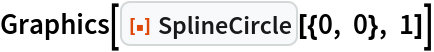Out=A 2D elliptical arc:

 In:=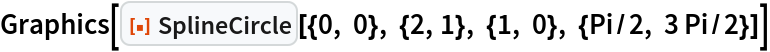Out=A 2D ellipse with semimajor axis in the direction {4, 1}:

 In:=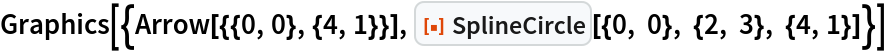Out=Differently styled ellipses:

 In:=Out=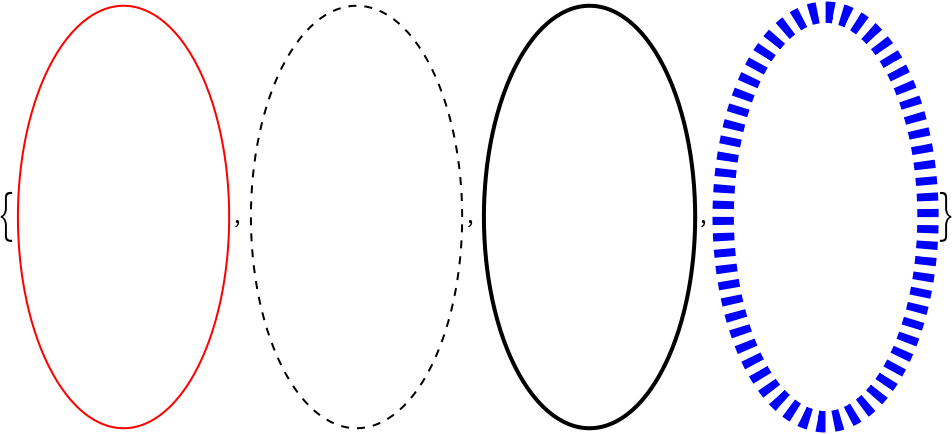A 3D circle:

 In:=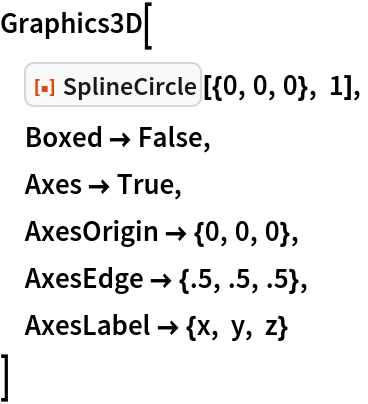Out=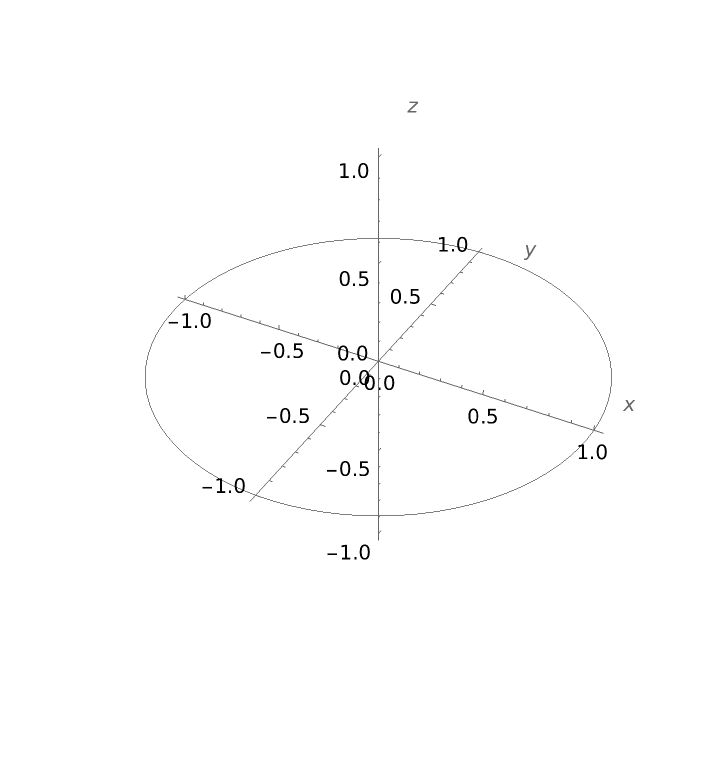A 3D ellipse:

 In:=Out=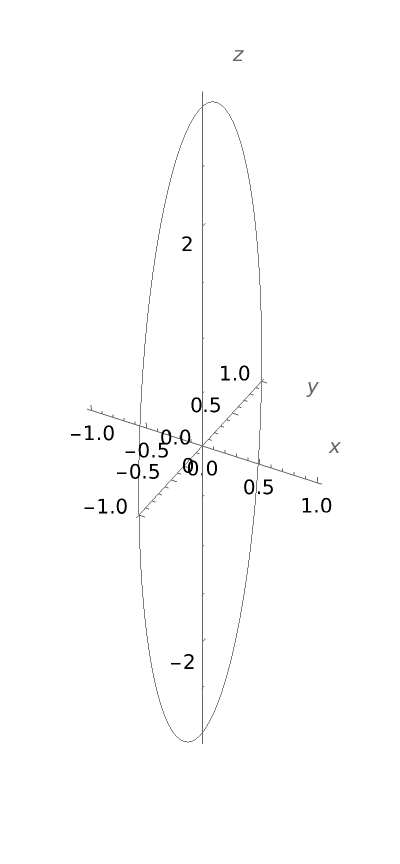A 3D elliptical arc:

 In:=Out=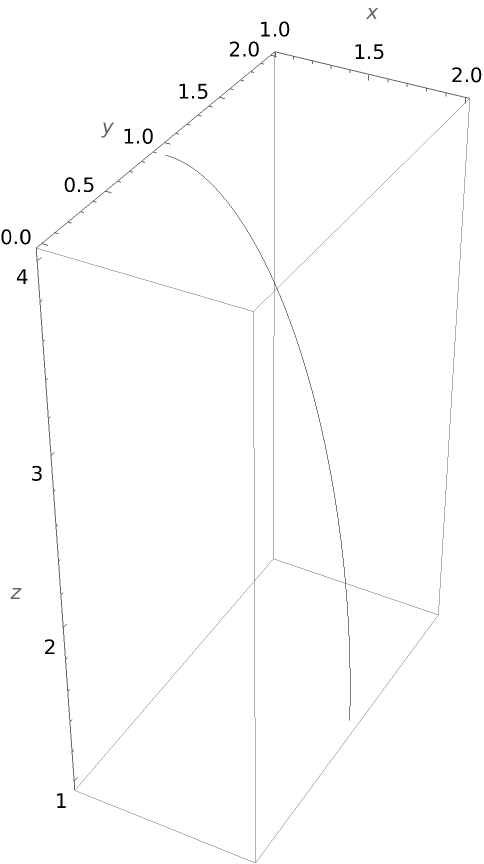### Scope (4)

Use SplineCircle as a FilledCurve specification:

 In:=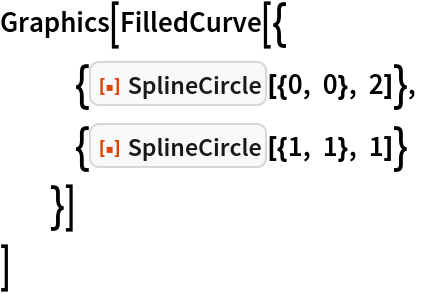Out=Use SplineCircle as a Tube curve specification:

 In:=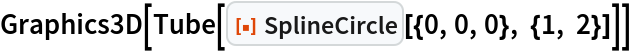Out=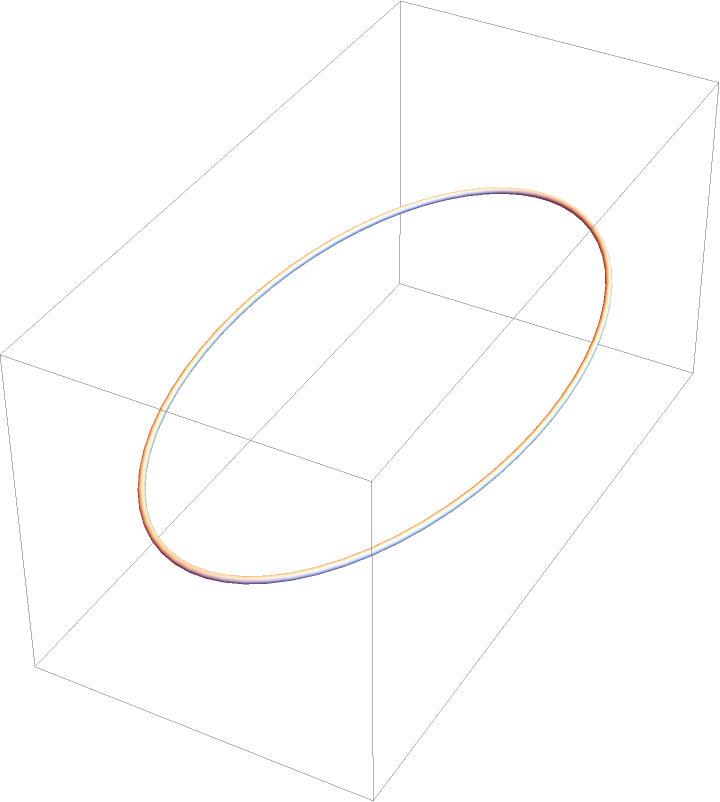Use SplineCircle as an Arrow curve specification:

 In:=Out=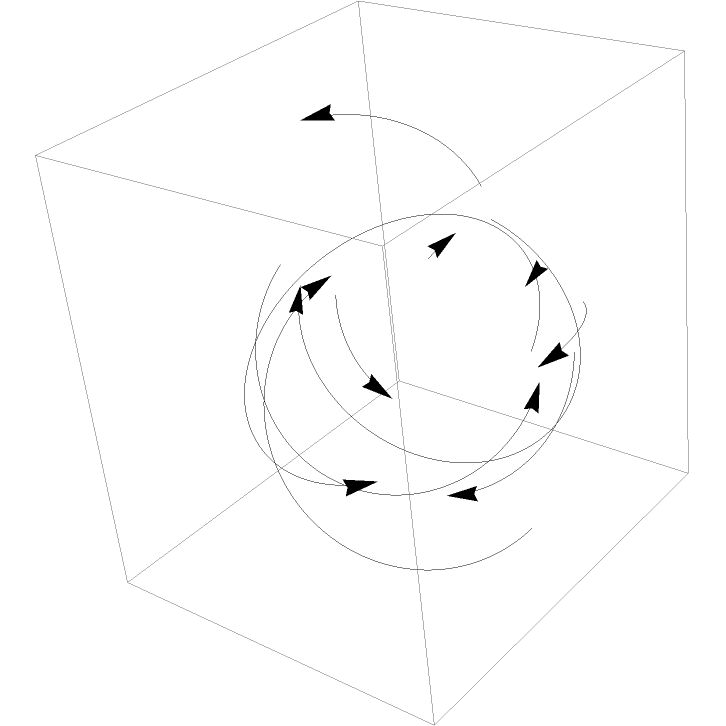Rendering of a fairly large number of circle tubes:

 In:=Out=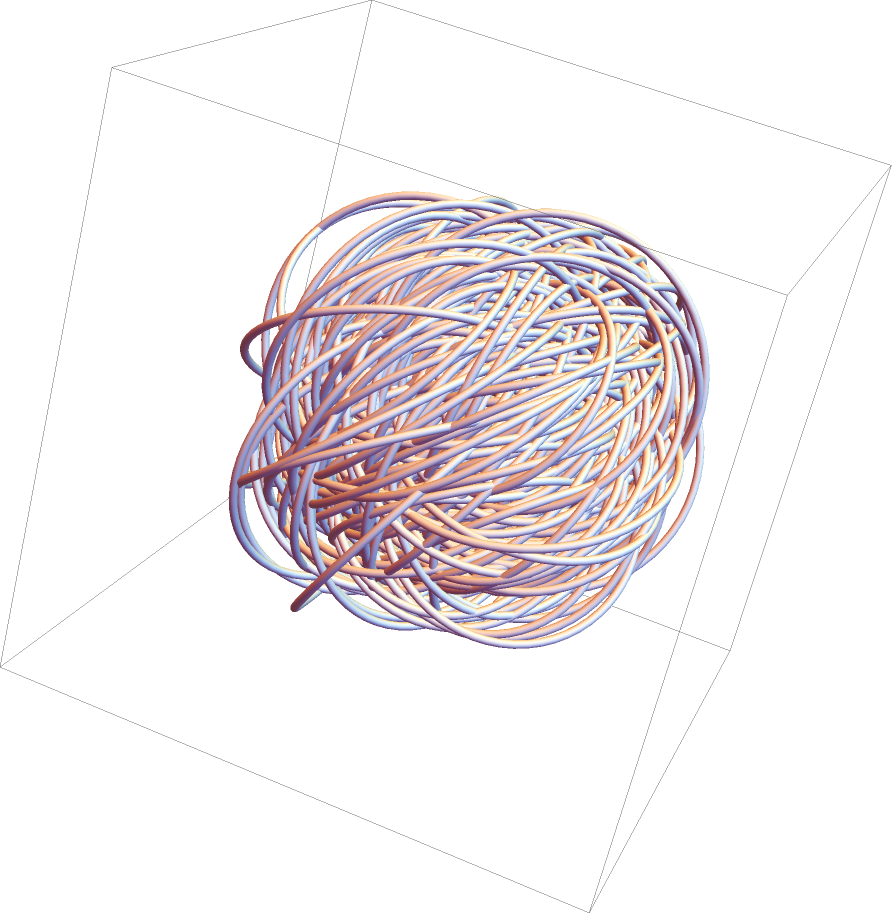### Properties and Relations (2)

A 2D SplineCircle with a non-default semimajor axis is equivalent to a geometric transformation of a Circle object:

 In:=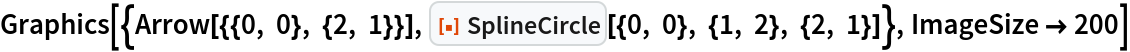Out=The Circle equivalent:

 In:=Out=### Possible Issues (3)

When only a symmetry axis {x,y,z} is specified, the largest component of the symmetry axis is computed, and the third coordinate starting with the largest component in a RHS is determined. Then the semimajor axis is assumed to be perpendicular to both the third component and the symmetry axis. For example, suppose the symmetry axis is:

 In:=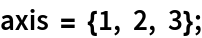The largest component is in the z direction, and so the third coordinate would be the y coordinate. Then the semimajor axis is assumed to be in the direction:

 In:=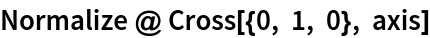Out=Check:

 In:=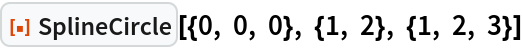Out=## Author Notes

I will add support for Scaled for both 2D and 3D graphics, and Offset and ImageScaled for 2D graphics.